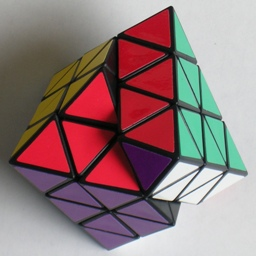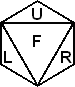# Face-turning OctahedronThis puzzle is an octahedron where all 8 faces can turn. The layers of two opposite faces are exactly the same thickness as the layer between them. The layer cuts therefore intersect each other in the centre of each triangular face. If the cuts were deeper so that there were no middle layers, then you would have the Skewb Diamond.

## The number of positions:

If you were to paint the puzzle with two colours, adjacent faces having different colours, then none of the moves will mix the colours. This shows that the vertex pieces have only two orientations, and the edges only one. Furthermore, the centres split in two sets that don't intermingle. This leaves us with 6 vertex pieces with 2 orientations, 12 edge pieces, and two sets of 12 centre pieces. This gives a combined upper bound of 6!·26·12!·12!2 arrangements. This limit is not reached because:

• Only an even number of vertex pieces are flipped (2)
• The vertex permutation is even (2)
• The edge permutation is even (2)
• The centres come in identical triplets (3!8)
• The orientation of the puzzle does not matter (12)

Note that the last factor is 12 rather than 24, since we fix the orientation of the puzzle by fixing one unique piece (a vertex or edge), which only has 12 possibilities. The total number of positions is therefore 6!·23·11!·12!2 / 3!8 = 31,408,133,379,194,880,000,000.

## Notation:Hold the puzzle with one face on top, its three corners pointing back, left, and right. Let U denote this top face. Let L, F, and R denote the left, front, and right visible faces. Note that F face shares a whole edge with U, whereas L and R each only share a vertex piece with U (and also a vertex with each other). The D face is the face on the bottom opposite the U face.
Clockwise turns of a face layer are denoted by the appropriate letter, anti-clockwise turns by the letter with an apostrophe (i.e. U', L', F', R', or D').

Phase 1: Solve the edges
This is quite straightforward. It is equivalent to solving a Dino Cube. The main difference is that you have to keep checking the colour arrangement on the vertex pieces to verify what the colour arrangement of the edges should be.

1. Choose a colour. Put the three edges with that colour together so that they have that colour together on one face. This is quite easy except that they must be placed in the correct order. Check that they are in the right order by looking at the colour arrangements on the three vertex pieces that they share the colour with.
2. With the three solved edges in the U face, solve the middle layer edges. You can do this without moving the three previously solved edges at all. Inserting an edge from the bottom layer into the middle layer is done as follows:
1. DL -> FR: Do the move sequence: R' D R
2. DR -> FL: Do the move sequence: L D' L'
You are free to turn the D layer, which allows you to insert any edge in the D layer into the middle layer. You can also use either sequence to remove any edge from the middle layer by displacing it by another. In this way all the middle layer edges can be solved.
Remember to keep checking the colour scheme is correct using the vertex pieces.
3. The last three edges are now trivially solved by at most a single turn of the D layer.

Phase 2: Solve the vertex pieces
This phase is equivalent to solving the edges of a Pyraminx.

1. Put all the vertex pieces in their correct locations (but not necessarily the correct orientation) by using the following three-cycles:
1. UR -> UL -> LR: Do the move sequence: R U' R' U
2. UB -> UR -> LR: Do the move sequence: R U R' U'
You can always hold the puzzle such that one of these sequences will correctly position at least one vertex and not disturb any already placed ones. Repeat this until they are all placed correctly.
2. You can flip an adjacent pair of vertex pieces using the following sequence:
UR+, UL+: Do the move sequence: R' U L' U' L U' R U, or alternatively do R U' R' U R' L R L'
If two opposite (non-adjacent) vertices need to be flipped, then you could do any move to make the two vertex pieces adjacent, flip them with the move sequence, and then undo the first move.

## Extra Notation:

The small letter r will denote the middle layer adjacent to the R face layer. The letter r will also mean a turn of that middle layer clockwise, as viewed from the R face. As usual, an anti-clockwise turn is denoted by an apostrophe (i.e. r').

Phase 3: Solve the centre pieces

1. Choose any unsolved centre piece. Hold the puzzle such this piece lies in the L face and that the face it belongs is the U face.
2. Turn the L face so that its incorrect centre piece (of the U face colour) lies on the right (in the r layer).
3. Turn the U face so that one of its incorrect centre pieces lies on the right (in the r layer). If you have a choice, prefer a centre piece of the L face colour over any others.
4. Do r U r' U' five times. This affects only centre pieces.
5. If necessary, turn the U face back the way it was, undoing the move from step c.
6. If necessary, turn the L face back the way it was, undoing the move from step b.
7. Repeat steps a-f until all the centres are correct.